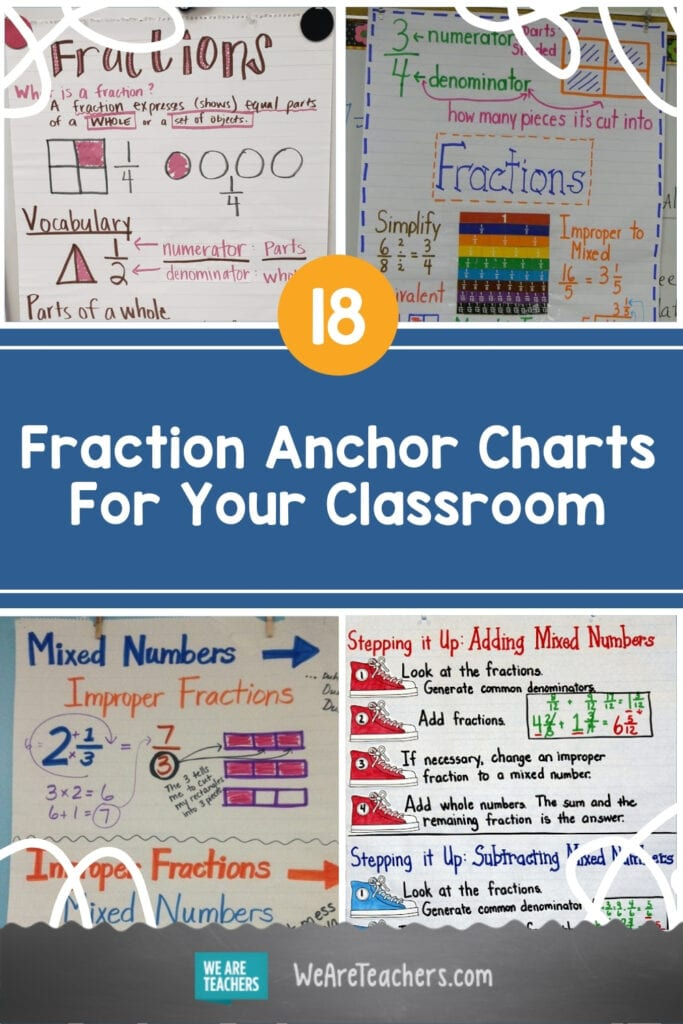Planning fraction lessons for your class? These fraction anchor charts can help support your lesson and reinforce student understanding. You’ll find examples on fraction vocabulary, comparing and simplifying, math operations, and mixed numbers below!

## 1. Learn the vocabulary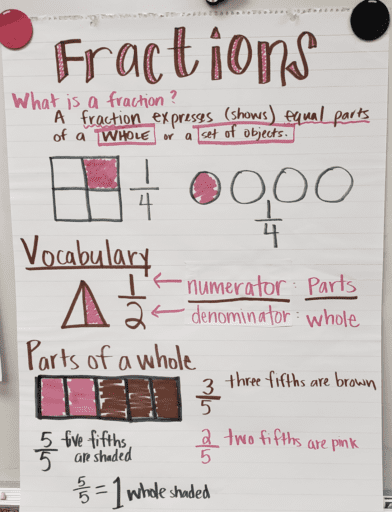First and foremost, help students understand fraction vocabulary, so the lesson runs smoothly.

Source: Liberty Pines

## 2. What is a fraction?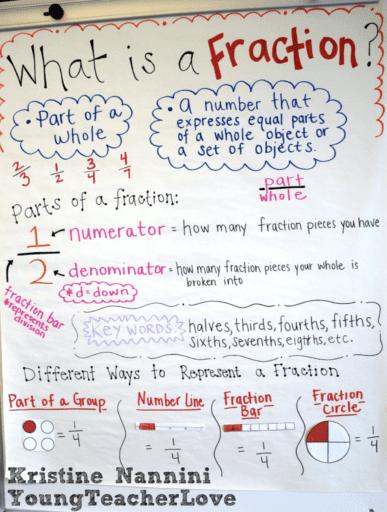This can be kept for students to reference throughout your fraction lessons.

Source: Young Teacher Love

## 3. Using a number line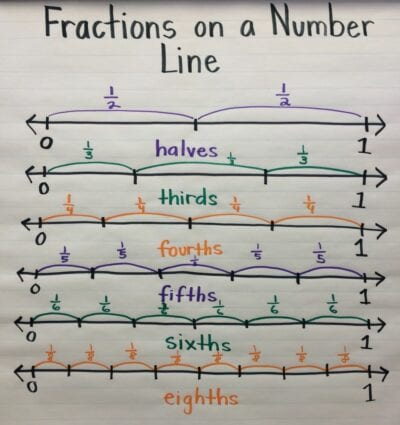Visualizing the parts of a whole that each fraction represents is possible using number lines.

Source: Mill Creek

## 4. Representing fractions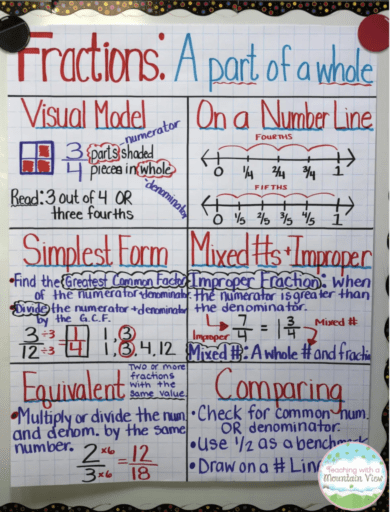Different variations of how to display and think about fractions give students multiple ways to grasp the concept.

Source: Teaching with a Mountain View

## 5. Comparing fractions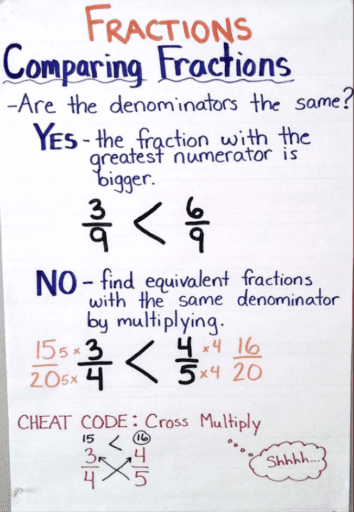Focus on denominators to compare fractions.

Source: One Stop Teacher Shop

## 6. Equivalent fractions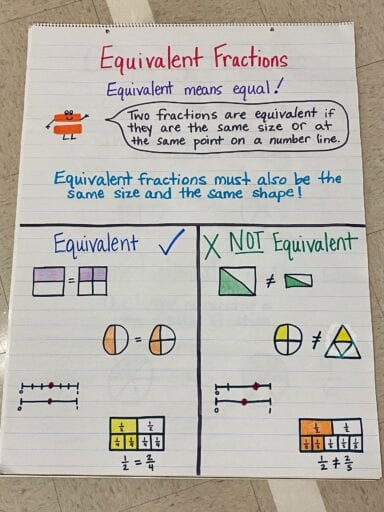Teaching equivalent fractions is fundamental before moving into using math operations with fractions.

Source: C.C. Wright Elementary

## 7. Proper and improper fractions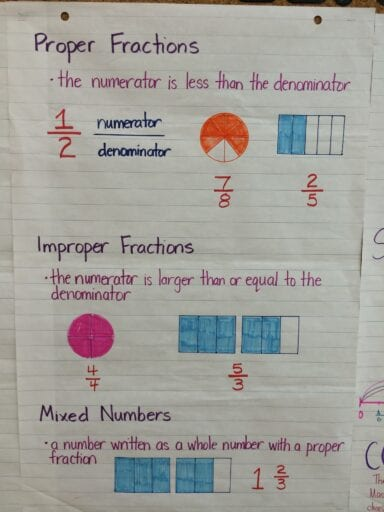Gain an understanding of proper versus improper fractions with pie pieces and building blocks.

Source: Mrs. Lee

## 8. Simplifying fractions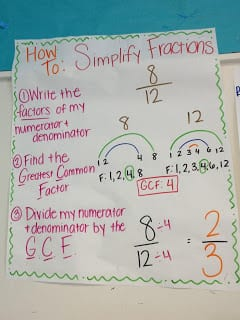Define and use the greatest common factor with this anchor chart.

Source: Teaching Coast 2 Coast

## 9. Display fraction concepts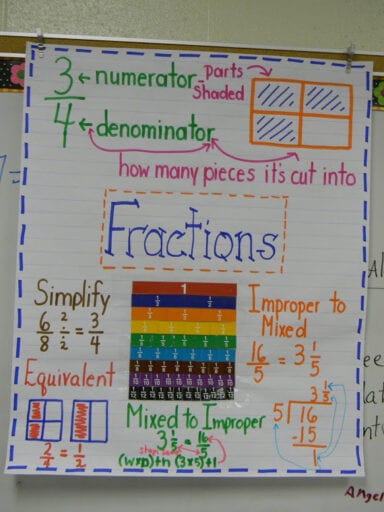Display multiple fraction concepts in one cohesive chart for a great student reminder.

Source: Teaching in High Heels

## 10. Making common denominators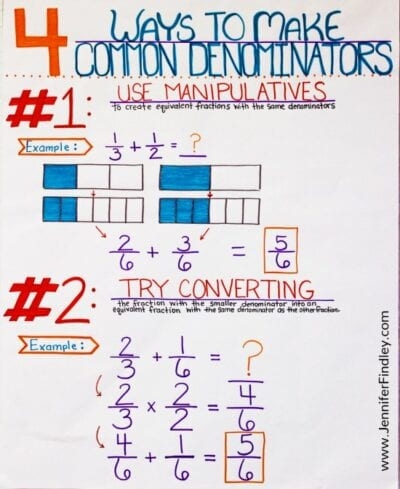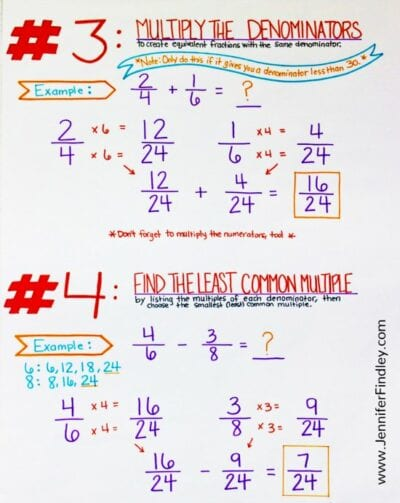These four options to make common denominators allow your students to find a method that works for them.

Source: Jennifer Findley

## 11. Steps of adding and subtracting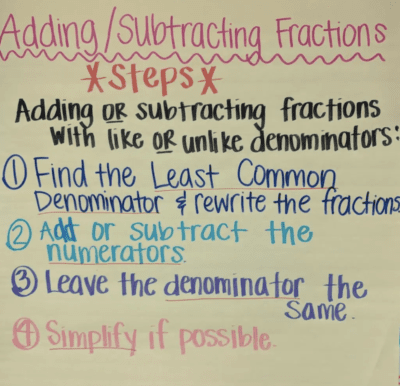Post this in the classroom to give students a 4-step process to follow when learning to add and subtract fractions.

Source: Life with Ones

## 12. Adding fractions with unlike denominators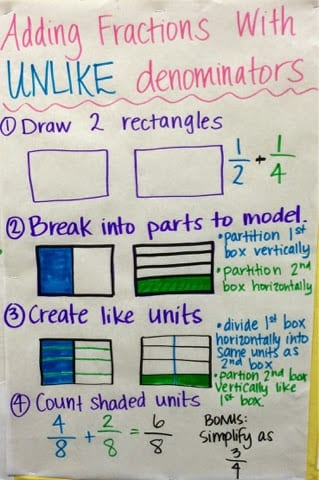Changing unlike denominators can be visualized with this block method.

Source: Mrs. Sandford

## 13. Subtracting fractions with unlike denominators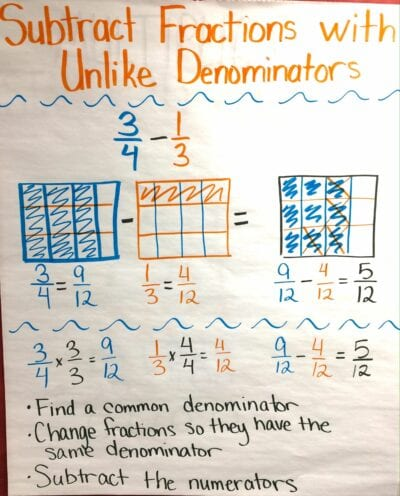Give these steps and visuals for subtracting with unlike denominators.

Source: Blend Space

## 14. Multiplying fractions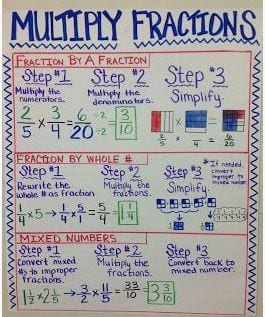Having steps gives an easy guide for students to follow along with as they implement the different types of numbers a fraction can be multiplied by.

Source: Mrs. Belbin

## 15. Dividing fractions with word problems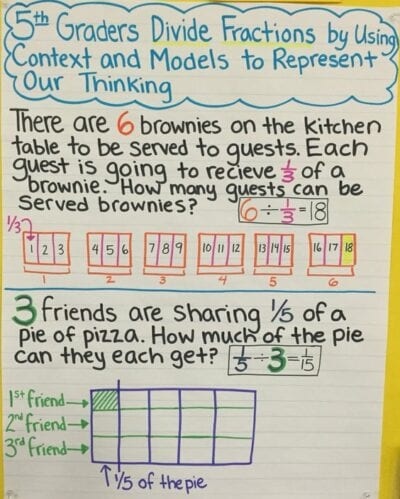Word problems create real-life scenarios for students to grasp division with fractions.

Source: Mrs. Doerre

## 16. What is a mixed number?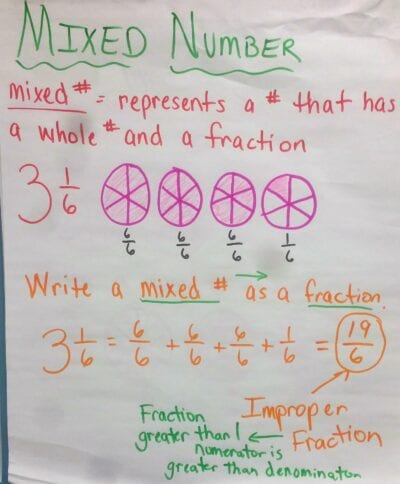Explain mixed numbers in relation to fractions.

Source: Kings Mountain

## 17. Mixed numbers and improper fractions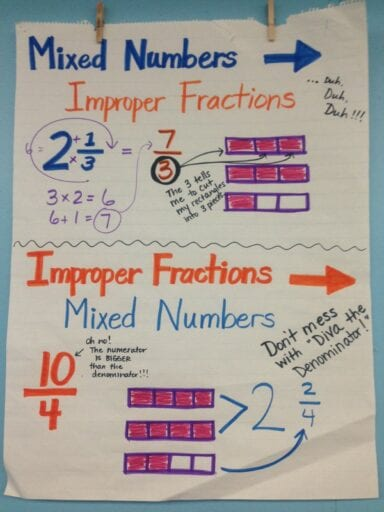Switching between mixed numbers and improper fractions is vital.

Source: thetaylortitans

## 18. Add and subtract mixed numbers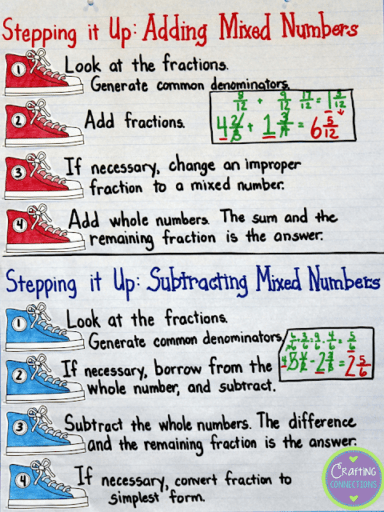Include mixed numbers in addition and subtraction with these fun “sneaker” steps.

Source: Crafting Connections

### Looking for more ways to teach fractions? Check out: